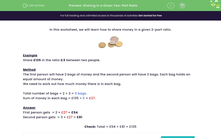# Share Quantities in a Given Two-Part Ratio

In this worksheet, students will share sums of money in the given two-part ratios.Key stage:  KS 3

Curriculum topic:   Ratio, Proportion and Rates of Change

Curriculum subtopic:   Use Ratio Notation

Popular topics:   Ratio worksheets

Difficulty level:#### Worksheet Overview

In this activity, we will learn how to share money in a given 2-part ratio.Example

Share £135 in the ratio 2:3 between two people.

Method

The first person will have 2 bags of money and the second person will have 3 bags. Each bag holds an equal amount of money.

We need to work out how much money there is in each bag.

Total number of bags = 2 + 3 = 5 bags.

Sum of money in each bag = £135 ÷ 5 = £27.

First person gets  2 × £27 = £54

Second person gets  3 × £27 = £81Check: Total = £54 + £81 = £135

Let's have a go at the questions now.

### What is EdPlace?

We're your National Curriculum aligned online education content provider helping each child succeed in English, maths and science from year 1 to GCSE. With an EdPlace account you’ll be able to track and measure progress, helping each child achieve their best. We build confidence and attainment by personalising each child’s learning at a level that suits them.

Get started